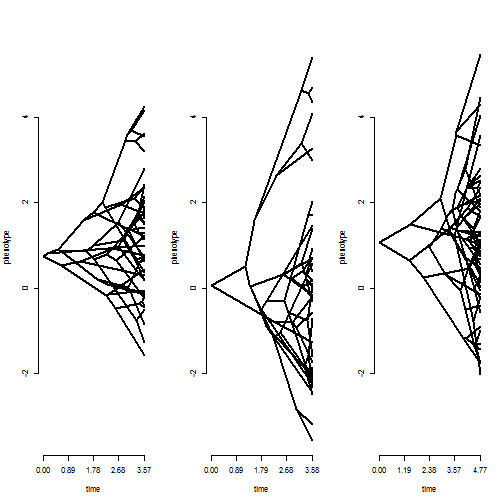## Saturday, September 23, 2017

### `AIC` method for `"ratebytree"` object class

I just pushed a few small fixes plus a new S3 `AIC` method for `"ratebytree"` class objects.

This is what the function looks like:

``````AIC.ratebytree<-function(object,...,k=2){
aic<-data.frame(AIC=c(k*object\$common.rate.model\$k-2*object\$common.rate.model\$logL,
k*object\$multi.rate.model\$k-2*object\$multi.rate.model\$logL),
df=c(object\$common.rate.model\$k,object\$multi.rate.model\$k))
} else rownames(aic)<-c("common-rate","multi-rate")
aic
}
``````

I'm not totally sure this is legal, but what the method does if multiple objects are supplied is that it assumes the common-rate model is the same for all supplied objects, and then it computes AIC values for only the multiple rate (or process) model for all subsequent objects.

Here's a demo using the same tree & data from earlier today:

``````library(phytools)
## our data:
par(mfrow=c(1,3))
nulo<-mapply(phenogram,tree=trees,x=x,MoreArgs=list(ylim=range(x),
ftype="off"))
````````````## fit two-rate model
fit.reg<-ratebytree(trees,x,regimes=c(1,2,1))
fit.reg
``````
``````## ML common-rate model:
##          s^2     a    a    a    k   logL
## value    1.2117  0.7489  0.058   1.0751  4   -223.603
## SE       0.1399  0.8292  1.1014  1.2046
##
## ML multi-rate model:
##          s^2  s^2  a    a    a    k   logL
## value    0.8862  2.1068  0.7489  0.058   1.0751  5   -217.4606
## SE       0.1195  0.4711  0.7091  1.4522  1.0302
##
## Likelihood ratio: 12.285
## P-value (based on X^2): 5e-04
##
## R thinks it has found the ML solution.
``````
``````## fit all rates different model
fit.all<-ratebytree(trees,x)
fit.all
``````
``````## ML common-rate model:
##          s^2     a    a    a    k   logL
## value    1.2117  0.7489  0.058   1.0751  4   -223.603
## SE       0.1399  0.8292  1.1014  1.2046
##
## ML multi-rate model:
##          s^2  s^2  s^2  a    a    a    k   logL
## value    0.6709  2.1068  1.0656  0.7489  0.058   1.0751  6   -216.0332
## SE       0.1342  0.4711  0.1946  0.617   1.4523  1.1297
##
## Likelihood ratio: 15.1397
## P-value (based on X^2): 5e-04
##
## R thinks it has found the ML solution.
``````
``````## now compute AIC scores:
AIC(fit.reg,fit.all)
``````
``````##                   AIC df
## common-rate  455.2061  4
## multi-rate:1 444.9211  5
## multi-rate:2 444.0664  6
``````

In this case, the all-rates-different model has the lowest AIC score, but the difference in fit between that & the two-rate model is quite small. Neat.